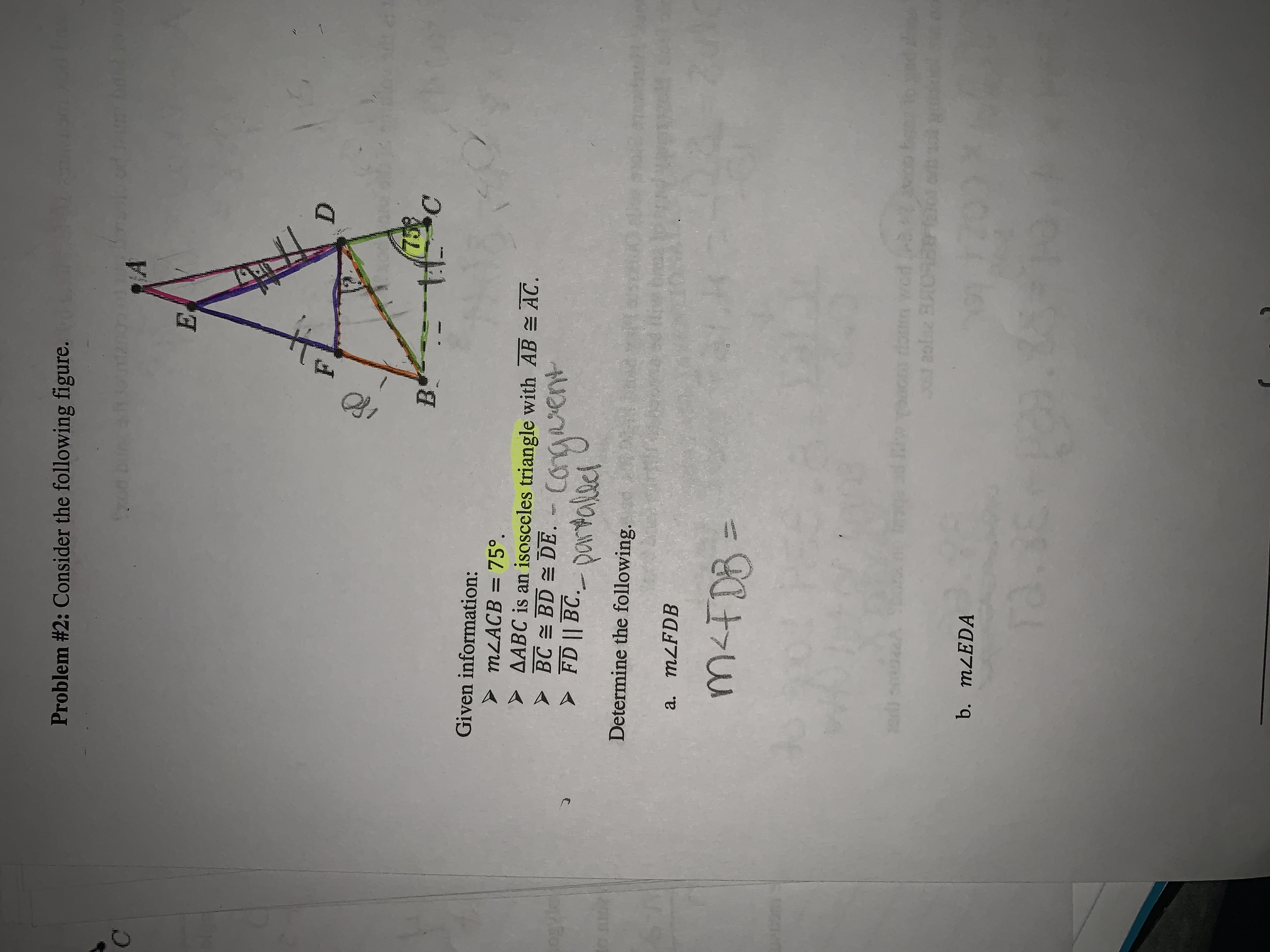# Problem #2: Consider the following figure.ro ufAfoxdD759CBGiven information:MLACB 75°AABC is an isosceles triangle with ABBC BD DEAC.CopartalectDetermine the following.FD I BC.ONtolci entগa. MLFDB10)oucdo cdE 20liz BUTCHb. MLEDATA.

Questionhelp_outlineImage TranscriptioncloseProblem #2: Consider the following figure. ro uf A foxd D 759 C B Given information: MLACB 75° AABC is an isosceles triangle with AB BC BD DE AC. Co partalect Determine the following. FD I BC.ONtolci ent গ a. MLFDB 10) oucdo cd E 20liz BUTCH b. MLEDA TA. fullscreen
check_circle

star
star
star
star
star
1 Rating
Step 1

In triangle BCD, BC=BD.

So, <BCD=<BDC=75

<DBC= 180-(75+75)= 30.

Step 2

FD || BC , so <FDB = <DBC . (Alternate angle)

So, <FDB= 30 degree

Step 3

Since triangle ABC is isosceles with AB=AC

So,  <ABC= < ACB = 75

...

### Want to see the full answer?

See Solution

#### Want to see this answer and more?

Solutions are written by subject experts who are available 24/7. Questions are typically answered within 1 hour.*

See Solution
*Response times may vary by subject and question.
Tagged in

### Other